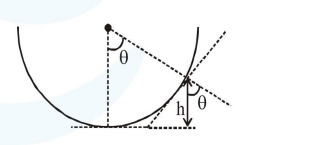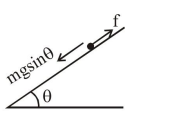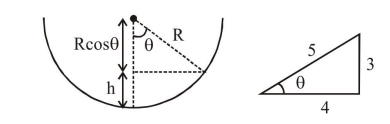# An insect is at the bottom of a hemispherical ditch of radius

Question:

An insect is at the bottom of a hemispherical ditch of radius $1 \mathrm{~m}$. It crawls up the ditch but starts slipping after it is at height $h$ from the bottom. If the coefficient of friction between the ground and the insect is $0.75$, then $\mathrm{h}$ is :

1. $0.80 \mathrm{~m}$

2. $0.60 \mathrm{~m}$

3. $0.45 \mathrm{~m}$

4. $0.20 \mathrm{~m}$

Correct Option: 4,

Solution:For balancing $m g \sin \theta=f$

$m g \sin \theta=\mu m g \cos \theta$

$\tan \theta=\mu$

$\tan \theta=\frac{3}{4}$$h=R-R \cos \theta$

$=\mathrm{R}-\mathrm{R}\left(\frac{4}{5}\right)=\frac{\mathrm{R}}{5}$

$\mathrm{h}=\frac{\mathrm{R}}{5}=0.2 \mathrm{~m}$

$\therefore$ correct option is $(4)$